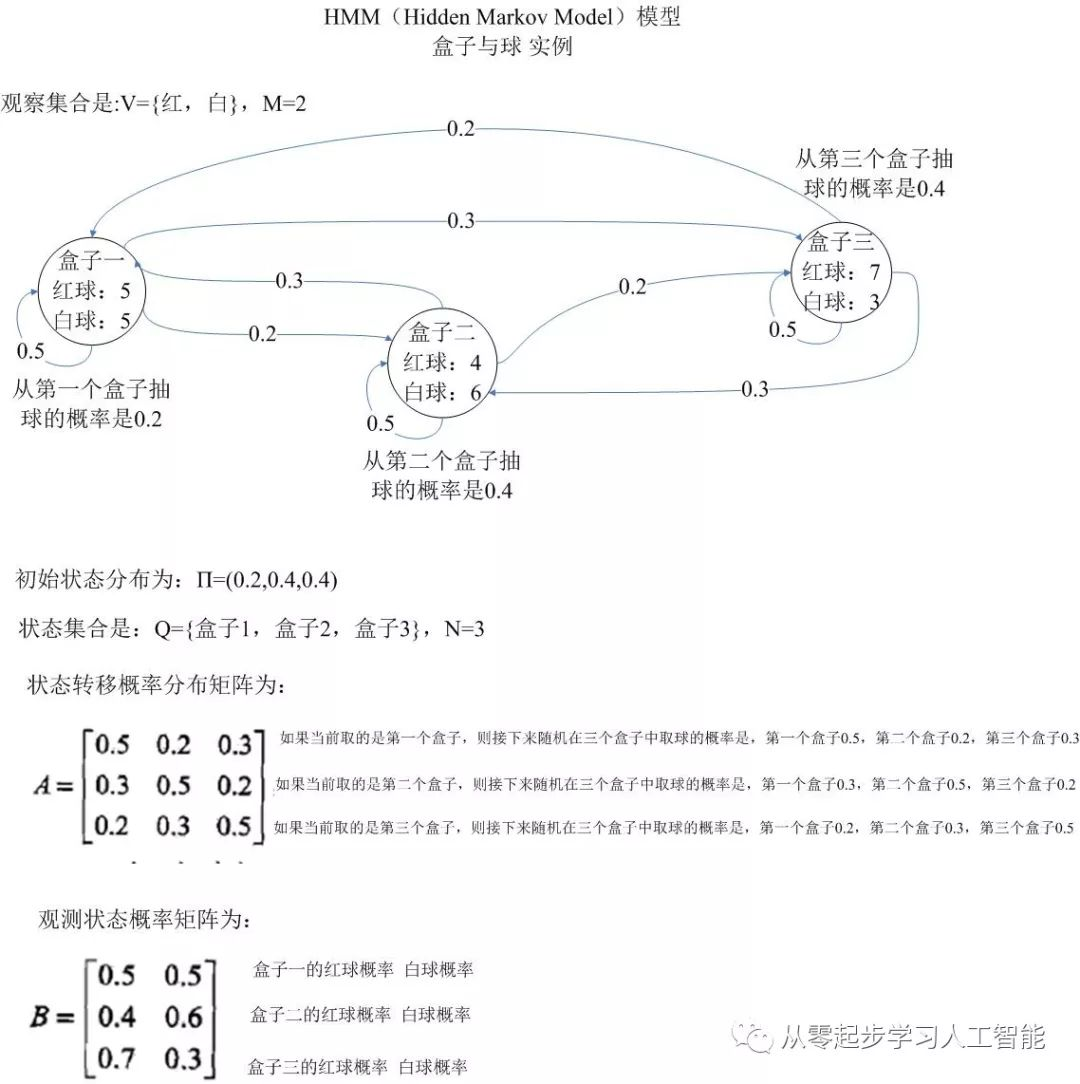## HMM（二）前向算法计算HMM观测序列的概率_段智华的博客-程序员秘密

沿用上一节中HMM模型盒子和球的例子，我们使用前向算法计算HMM观测序列的概率。• 在第一个时刻取到的是红色球：

``α1(1)=π1b1(o1)=0.2×0.5=0.1 ``

π1指π=（0.2，0.4，0.4）的第一个元素0.2，从第一个盒子中抽到球的概率是0.2；b1(o1)其中的o1是观测序列的第一个时刻取到的红球，b1(o1)从观测状态概率矩阵b1盒子一种取红球的概率是0.5。

``α1(2)=π2b2(o1)=0.4×0.4=0.16 ；``

π2指π=（0.2，0.4，0.4）的第2个元素0.4，从第2个盒子中抽到球的概率是0.4；b2(o1)其中的o1是观测序列的第一个时刻取到的红球，b2(o1)从观测状态概率矩阵b2盒子一种取红球的概率是0.4。

隐藏状态是盒子3的概率为：

``α1(3)=π3b3(o1)=0.4×0.7=0.28；``

π3指π=（0.2，0.4，0.4）的第2个元素0.4，从第3个盒子中抽到球的概率是0.4；b3(o1)其中的o1是观测序列的第一个时刻取到的红球，b3(o1)从观测状态概率矩阵b3盒子一种取红球的概率是0.7。

• 在第2个时刻取到的是白色球：以下序号i从i=1至i=3 求和

``α2(1)=[∑α1(i)ai1] b1(o2)=[0.1∗0.5+0.16∗0.3+0.28∗0.2]×0.5=0.077``

α1(i) 是第一个时刻隐藏状态为第i个盒子的概率，ai1是状态转移概率分布矩阵中取到第i个盒子以后接下来再取到第1个盒子的概率，然后将α1(i)ai1做点积计算[∑α1(i)ai1]，b1(o2)是观测状态概率矩阵中第一个盒子取到白球（第二个时刻取到的是白球）的概率为0.5

``α2(2)=[∑α1(i)ai2] b2(o2)=[0.1∗0.2+0.16∗0.5+0.28∗0.3]×0.6=0.1104``

α1(i) 是第一个时刻隐藏状态为第i个盒子的概率，ai2是状态转移概率分布矩阵中取到第i个盒子以后接下来再取到第2个盒子的概率，然后将α1(i)ai2做点积计算[∑α1(i)ai2]，b2(o2)是观测状态概率矩阵中第2个盒子取到白球（第二个时刻取到的是白球）的概率为0.6

``α2(3)=[∑α1(i)ai3] b3(o2)=[0.1∗0.3+0.16∗0.2+0.28∗0.5]×0.3=0.0606``

α1(i) 是第一个时刻隐藏状态为第i个盒子的概率，ai3是状态转移概率分布矩阵中取到第i个盒子以后接下来再取到第3个盒子的概率，然后将α1(i)ai3做点积计算[∑α1(i)ai3]，b3(o2)是观测状态概率矩阵中第3个盒子取到白球（第二个时刻取到的是白球）的概率为0.3

• 在第3个时刻取到的是红色球：以下序号i从i=1至i=3 求和

``α3(1)=[∑α2(i)ai1] b1(o3)=[0.077∗0.5+0.1104∗0.3+0.0606∗0.2]×0.5=0.04187``

``α3(2)=[∑α2(i)ai2] b2(o3)=[0.077∗0.2+0.1104∗0.5+0.0606∗0.3]×0.4=0.03551``

``α3(3)=[∑α3(i)ai3] b3(o3)=[0.077∗0.3+0.1104∗0.2+0.0606∗0.5]×0.7=0.05284``

``P(O|λ)=∑α3(i)=0.13022``

### nosql数据库中之redis数据库一揽子方案_gsdgsdfs的博客-程序员秘密

nosql数据库中之redis数据库一揽子方案

### IIS部署web服务_iis管理器没有部署怎么办_伤心小魔剑的博客-程序员秘密

1.安装IIS2.在IIS中添加该服务的站点3.启用文件浏览功能4.设置应用程序5.完工，检查。6.使用web服务我就不啰嗦